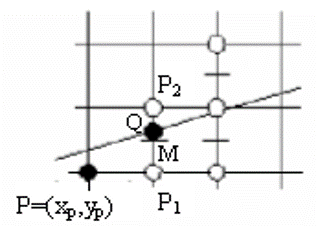中点画线法

基本推导

$M$$P_1$$P_2$ 的中点，易知 $M$ 的坐标为$(x_p+1,y_p+0.5)$

$Q$ 为理想直线与垂直线 $x=x_p+1$ 的交点。$F(x,y)=ax+by+c=0$

$a=y_0-y_1$
$b=x_1-x_0$
$c=x_0y_1-x_1y_0$$y=\left\{\begin{array}{lcl} y+1 &{d<0}\\ y &{d \geq 0} \end{array} \right.$

$d<0$ 时，说明 $M$ 点在直线的下方，那么直线离 $P_2(x_p+1,y_p+1)$ 点更近，所以下一个逼近于直线的点应该取 $P_2(x_p+1,y_p+1)$ ，即 $y=y+1$，反之，当 $d>0$ 时，说明 $M$ 点在直线的上方，那么直线离 $P_1(x_p+1,y_p$ 点更近，所以下一个逼近于直线的点应该取 $P_1$ ，即 $y$ 不变。当 $d=0$ 时说明 M 点在直线上，那么取 $P_1(x_p+1,y_p)$$P_2(x_p+1,y_p+1)$皆可。由于假设 $|k|<1$，所以 $x$ 每次都增加 1。

递推误差项$d$

$d\geq0$时：

$d_1=F(x_p+2,y_p+0.5)=a(x_p+2)+b(y_p+0.5)+c=a(x_p+1)+b(y_p+0.5)+c+a=d+a$

$d<0$ 时：

$d_1=F(x_p+2,y_p+1.5)=a(x_p+2)+b(y_p+1.5)+c=a(x_p+1)+b(y_p+0.5)+c+a+b=d+a+b$

$d_n=\left\{\begin{array}{lcl} d_{n-1}+a+b &{d<0}\\ d_{n-1}+a &{d \geq 0} \end{array} \right.$

中点画线算法公式

（1） 输入直线的起始和终止端点 $P_0 (x_0 ,y_0)$$P_1 (x_1 ,y_1)$

（2） 计算初始值$a= y_0 - y_1 ，b= x_1 - x_0 ，c= x_0 y_1 - x_1 y_0 ,d=a+0.5*b,x=x_0 ,y=y_0$

（3） 绘制点$(x,y)$

（4） 判断 $d$ 的符号。若 $d<0$，则$(x,y)$ 更新为 $(x+1,y+1)$$d$ 更新为 $d+a+b$；否则$(x,y)$ 更新为$(x+1,y)$$d$ 更新为 $d+a$

（5） 当直线没有绘制完成时，跳转到步骤（3）执行，否则算法结束。

优化中点画线算法

（1） 输入直线的起始和终止端点 $P_0 (x_0 ,y_0)$$P_1 (x_1 ,y_1)$
（2） 计算初始值$a= y_0 - y_1 ，b= x_1 - x_0 ，c= x_0 y_1 - x_1 y_0 ,d=2*a+b,x=x_0 ,y=y_0 ,d_1=2*a, d_2=2*(a+b)$
（3） 绘制点$(x,y)$
（4） 判断 $d$ 的符号。若 $d<0$，则$(x,y)$ 更新为 $(x+1,y+1)$$d$ 更新为 $d+d_2$；否则$(x,y)$ 更新为$(x+1,y)$$d$ 更新为 $d+d_1$
（5） 当直线没有绘制完成时，跳转到步骤（3）执行，否则算法结束。

总结

（1） 不必计算直线的斜率，因此不必除法运算；
（2） 不用浮点数，只用整数；
（3） 只有加法和乘 2 运算，在计算机内部用位移操作实现即可，因此效率很高。